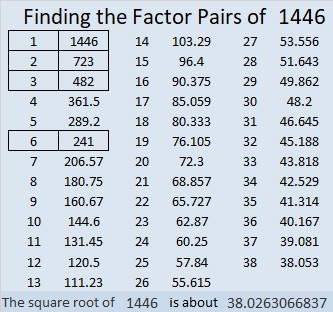# 1446 Peppermint Stick

Red and green striped peppermint sticks are often seen in stores and homes in December. Can you lick this peppermint stick puzzle or will you let it lick you?The puzzle number was 1446. Here are a few facts about that number:

• 1446 is a composite number.
• Prime factorization: 1446 = 2 × 3 × 241
• 1446 has no exponents greater than 1 in its prime factorization, so √1446 cannot be simplified.
• The exponents in the prime factorization are 1, 1, and 1. Adding one to each exponent and multiplying we get (1 + 1)(1 + 1)(1 + 1) = 2 × 2 × 2 = 8. Therefore 1446 has exactly 8 factors.
• The factors of 1446 are outlined with their factor pair partners in the graphic below.1446 is also the hypotenuse of a Pythagorean triple:
720-1254-1446 which is 6 times (120-209-241)